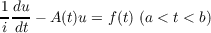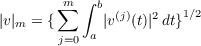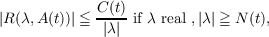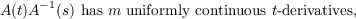#### Vol. 16, No. 2, 1966

 Download this articleFor screen For printingRecent Issues Vol. 311: 1 Vol. 310: 1  2 Vol. 309: 1  2 Vol. 308: 1  2 Vol. 307: 1  2 Vol. 306: 1  2 Vol. 305: 1  2 Vol. 304: 1  2Online Archive Volume: Issue:The Journal Subscriptions Editorial Board Officers Contacts Submission Guidelines Submission Form Policies for Authors ISSN: 1945-5844 (e-only) ISSN: 0030-8730 (print) Special Issues Author Index To Appear Other MSP Journals
Differentiability of solutions of ordinary differential equations in Hilbert space

### Avner Friedman

Vol. 16 (1966), No. 2, 267–271
##### Abstract

Consider the differential equation(1.1)

where u(t), f(t) are elements of a Hilbert space E and A(t) is a closed linear operator in E with a domain D(A) independent of t and dense in E. Denote by Cm(a,b) the set of functions v(t) with values in E which have m strongly continuous derivatives in (a,b). Introducing the norm(1.2)

where |v(t)| is the E-norm of v(t), we denote by Hm(a,b) the completion with respect to the norm (1.2) of the subset of functions in Cm(a,b) whose norm is finite. Set Hm = Hm(−∞,) and denote by H0m the subset of functions in Hm which have compact support. The solutions u(t) of (1.1) are understood in the sense that u(t) H1(a,b) for any a < a< b< b.

Theorem 1. Assume that, for each a < t < b, the resolvent R(λ,A(t)) = (λA(t))1 of A(t) exists for all real λ, |λ|N(t), and that(1.3)

where N(t), C(t) are constants. Assume next that for each s (a,b), A1(s) exists and(1.4)

for a < t < b, where m is any integer 1. If u is a solution of (1.1) and if f Hm(a,b), then u Hm+1(a,b) for any a < a< b< b.

Theorem 2. If the assumptions of Theorem 1 hold with m = , if A(t)A1(s) is analytic in t(a < t < b) for each s (a,b), and if f(t) is analytic in (a,b), then u(t) is also analytic in (a,b).

Primary: 34.95
##### Milestones